# Diode Clamper Circuits

Published  August 28, 2019   0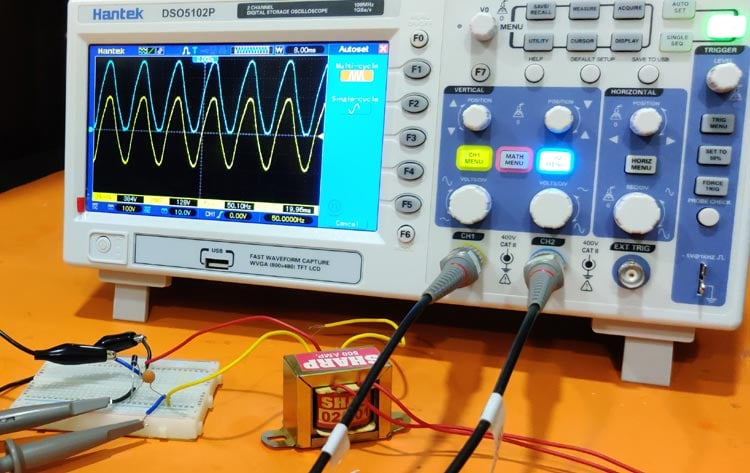Previously we learned about Clipper Circuits, which are used to clip off the positive or negative part of the Alternating waveform. Today we will learn about Clamper circuits which are used to clamp the DC level of the output signal without distorting the waveform i.e. they are Level Shifter circuits. It can be designed using capacitor, diode and resistor. The difference between a clipper and a clamper is that the clipper circuit changes the shape of the waveform but clamper just manipulates the DC level of output signal.

While choosing the resistor and capacitor you must be aware about the discharging time of the capacitor as it maintains the time period of the waveform. It must be quite larger than the half of the time period so that the capacitor discharges slowly. The electrolytic capacitor should not be used in clamper circuits because they charge and discharge slowly. The discharging time () can be calculated using the below formula:

`t (Tau) = RC`

Where R is the resistance used in the circuit and C is the capacitance of the capacitor.

There are mainly three types of clamper circuits based upon the clamping:

1. Positive Clamper
2. Negative Clamper
3. Biased Clamper

### Positive Clamper

When the negative cycle clamps/shifts above the zero voltage level, then the clamper circuit is called as Positive Clamper because the whole signal is shifted to the positive side. It’s a really simple circuit to design, you just need to follow the circuit diagram below: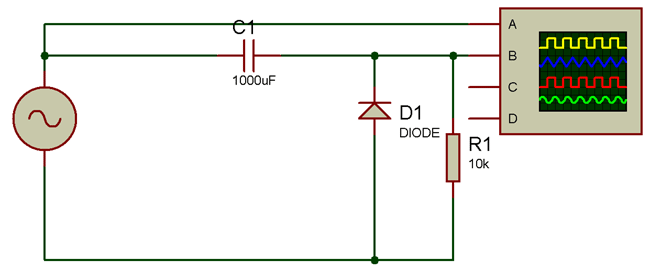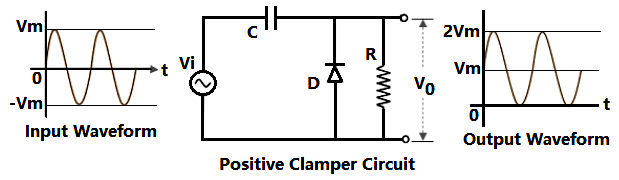First connect the transformer’s 12V (AC source) pin to the capacitor and then connect diode’s negative terminal to the other terminal of capacitor and positive terminal to 0V pin of the transformer. Now connect a 10K resistor in parallel to the diode. Connect the channel A of oscilloscope to the input side and channel B to the output side as shown in figure. Now you are ready to go. Turn ON the transformer and oscilloscope and adjust both of the channels to 0V line and you’ll see that the channel B is shifted upwards as shown below: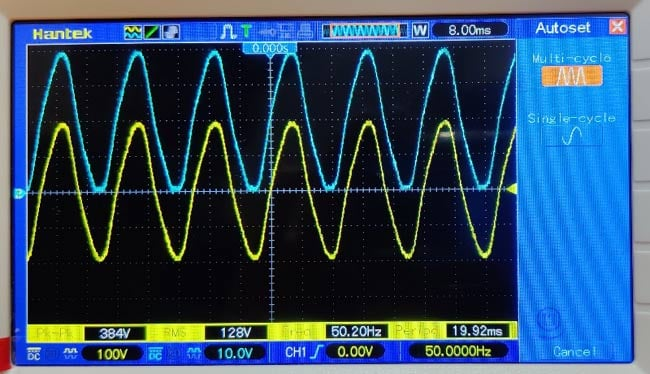During the first positive half cycle the diode is reverse biased and the capacitor does not get charged at the peak value. But during the negative half cycle the diode gets forward biased and the capacitor is charged at its peak value Vm. And the output voltage becomes:

`Vo= Vi + Vm`

Here Vi is the input voltage, Vo is the output voltage, and Vm is the maximum voltage to which capacitor gets charged. Hence the output gets shifted by + Vm level. This shifting solely depends upon the charge stored by the capacitor.

### Negative Clamper

When the positive cycle clamps/shifts below the zero voltage level, then the clamper circuit is called as Negative Clamper because the whole signal is shifted to the negative side. The circuit diagram to build an Negative clamper is shown below: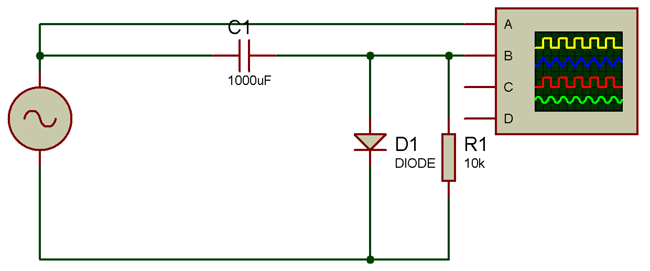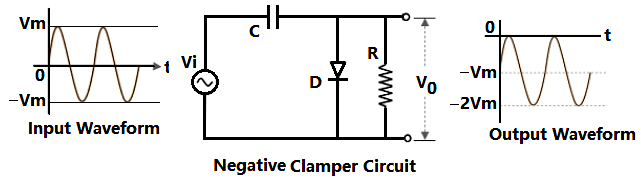First connect the transformer’s 12V (AC source) pin to the capacitor and then connect diode’s positive terminal to the other terminal of capacitor and negative terminal to 0V pin of the transformer. Now connect a 10K resistor in parallel to the diode. Connect the channel A of oscilloscope to the input side and channel B to the output side as shown in figure. Now you are ready to go. Turn ON the transformer and oscilloscope and adjust both of the channels to 0V line and you’ll see that the channel B is shifted downwards as shown in the figure below. The channel A is yellow colored and channel B is blue colored.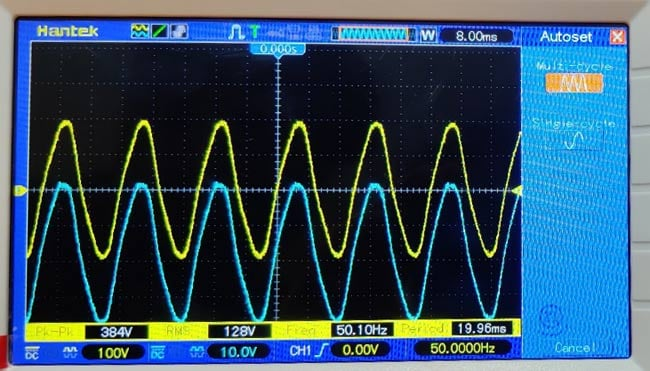During the first positive half cycle the diode is forward biased and the capacitor get charged at the peak value Vm and during the negative half cycle the diode gets reverse biased and acts as a open circuit. So, the output voltage becomes:

`Vo= Vi + Vm`

Here Vi is the input voltage, Vo is the output voltage, and Vm is the maximum voltage to which capacitor gets charged. Hence the output gets shifted by –Vm level because it is the negative voltage. This shifting solely depends upon the charge stored by the capacitor.

### Biased Clamper

A biased clamper is nothing different than positive and negative clampers discussed earlier. It just consists of a bias voltage with diode.

So, if you connect the bias voltage with positive clamper then it just gets added with output voltage and it will shift to more positive level as the bias voltage.

And if you connect the bias voltage with negative clamper then it just gets added with output voltage and it shifts to more negative level as the bias voltage.

But remember if you connect a negative bias voltage with positive clamper then instead of shifting to the positive level it will shift to some negative level because it will get subtracted from output voltage.

And if you connect a positive bias voltage with negative clamper then instead of shifting to the negative level it will shift to some positive level because it will get subtracted from output voltage.

We’ve designed a positive clamper with positive bias voltage below.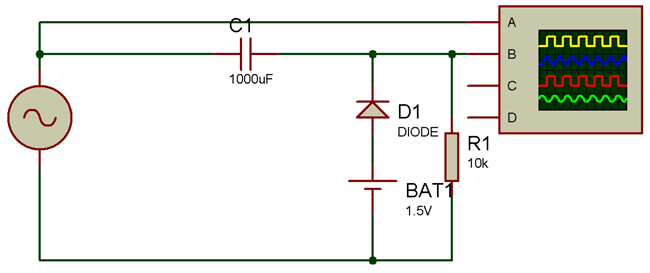The negative clamper can also be designed in the same way just by reversing the diode and bias voltage.

Bias voltage can be of any value but remember it should not be greater than or equal to the input voltage because in that case either you will not get any output, or the clamping may get reversed.